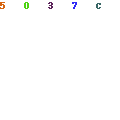# Introduction to Adaptive Boosting ClassifierI am just finishing writing my master’s thesis, whose subject is recognizing epileptic states based on the EEG signal using the ensemble classifiers. One of the ensemble classifiers that I used in my research was Adaptive Boosting Classifier. I decided to write an article about it. This will be a brief theoretical introduction to Adaptive Boosting Classifier and an example of its implementation in Python using the Scikit-learn library.

Theoretical introduction

Adaptive Boosting Classifier is an ensemble classifier developed by Yoav Freund and Robert Schapire. This algorithm works by creating a prediction model in the form of a set of weak models. It requires specifying a set of weak learners before actually starting it. The weight of each model is determined based on whether it correctly predicted the sample or not. In a situation where the learner has predicted wrong, his weight is slightly reduced. The whole process is carried out until convergence.

The algorithm can be presented in the following steps:

1. Assignment of all samples w^{(i)} the initial value of \frac{1}{m}

2. Learn the first predictor and calculate the weighted error rate r_1 for it (where \widehat{y}_j^{(i)} is the j-th forecast for the i-th case):

3. Calculation of the predictor weight \alpha_j, where $\eta$ is the learning factor:

4. Updating weights – strengthening of incorrectly classified samples:

5. Normalization of all weights of examples.

6. Learning a new predictor using updated weights and repeating the whole process until the intended number of predictors is achieved or satisfactory results are obtained.

7. Algorithm forecasting by checking the predictions of all predictors and their weights using the w^{(i)} factor, and then selecting the predicted class by majority vote:

Example in Python

If you want to use this algorithm in Python, you can do that with the sklearn library. Just import the following method:

from sklearn.ensemble import AdaBoostClassifier

Let me leave part of the implementation responsible for preparing the training and test set. I will proceed to use the classifier:

abc = AdaBoostClassifier()
abc.fit(x_train, y_train.ravel())
predictions = abc.predict(x_test)

The library allows you to set certain parameters for the classifier, such as the depth of individual estimators or tolerance for premature stopping of the process. All information can be found in the documentation.

Summary

I wanted to introduce you to Adaptive Boosting Classifier. The subject of ensemble classifiers is very extensive and interesting. The classifier which I presented today is one of the pillars. I really recommend that you delve deeper into this. I also encourage you to read my latest article about Cohen’s kappa coefficient.

Sources

1. Aurelien Geron, Hands-on Machine Learning with Scikit-Learn, Keras, and TensorFlow: Concepts, Tools, and Techniques to Build Intelligent Systems, O’Reilly Media, Inc, USA, 2019
Scroll to top